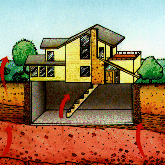Radon is a gas produced by the radioactive decay of the element radium. Radioactive decay is a natural, spontaneous process in which an atom of one element decays or breaks down to form another element by losing atomic particles (protons, neutrons, or electrons). When solid radium decays to form radon gas, it loses two protons and two neutrons. ...Biology

### Microarrays: Chipping Away At The Mysteries Of Science And Medicine

With only a few exceptions, every cell of the body contains a full set of chromosomes and identical genes. Only a fraction of these genes are turned on, however, and it is the subset that is ... Continue reading

Astronomy

Take a piece of paper. Make a little wad. If you're a kid, spit on it. Put it in a straw and blow hard. If your teacher sends you to the principal's office, here's your excuse: you were making a model ... Continue reading

Physics

### Newton's Three Laws of Motion

The motion of an aircraft through the air can be explained and described by physical principals discovered over 300 years ago by Sir Isaac Newton. Newton worked in many areas of mathematics and ... Continue reading

Mathematics

### Leaps and Bounds

Leap years are years with 366 days, instead of the usual 365. Leap years are necessary because the actual length of a year is 365.242 days, not 365 days, as commonly stated. Basically, leap years ... Continue reading

## Fibonacci Patterns In Nature?Often it takes a second look to see how mathematical numbers and patterns fit into the natural world. Numbers, after all, are manmade. However some very interesting number patterns underlie some natural systems in a surprising way. One such number pattern is called the Fibonacci Series, where each subsequent number is the sum of the two preceding numbers. For example 0, 1, 1, 2, 3, 5, 8, 13, 21... The next in the series is 13 + 21 = 34, and so on.

The trick with mathematical patterns is to see them in the first place, and it wasn't until the 13th century that Leonard Fibonacci, of Pisa, Italy, first described this series in a book on calculations. Since that time, scientists have found the Fibonacci Series in many different places. In the plant kingdom petals on flowers and leaves on stems are often arranged in groups of 3s, 5s and 8s. Pine cones, too, show the same series. In the animal kingdom, the spirals of seashells can be found following the series, as can genealogical patterns of honey bees.

### Science Articles: